• Matlab多种图像边缘检测分割处理-多种图像边缘检测分割处理.rar 是一些边缘检测算子，用这些算子实现图像边缘检测的程序
• 采用五种边缘检测算子对数字图像开展了检测，并进行了比较与分析 . 探究了阈值对图像边缘效果的影响 . 分析了不同算法在边缘的完整性、连续性、对噪声的敏感性等方面表现出的不一样的特性 .
• Hough线检测 问题1：对作业一中边缘检测的结果，进行Hough线检测。 问题2：调节参数，提取较长的边界。
• 采用阈值处理方法进行图像分割 实现直方图阈值法，具体方法为采用灰度直方图求双峰或多峰，选择两峰之间的谷底作为阈值，将图像转换为2值图像
• matlab边缘检测和图像分割 图像和算法等领域有多年研究项目经验；指导发...matlab边缘检测和图像分割

图像和算法等领域有多年研究和项目经验；指导发表科技核心期刊经验丰富；多次指导数学建模爱好者参赛。宋星星

¥12.00

立即订阅

扫码下载「CSDN程序员学院APP」，1000+技术好课免费看
APP订阅课程，领取优惠，最少立减5元 ↓↓↓订阅后：请点击此处观看视频课程

视频教程-matlab边缘检测和图像分割-Matlab
学习有效期：永久观看
学习时长：101分钟
学习计划：2天
难度：低

「口碑讲师带队学习，让你的问题不过夜」讲师姓名：宋星星
工程师
讲师介绍：图像和算法等领域有多年研究和项目经验；指导发表科技核心期刊经验丰富；多次指导数学建模爱好者参赛。
☛点击立即跟老师学习☚

「你将学到什么？」

matlab边缘检测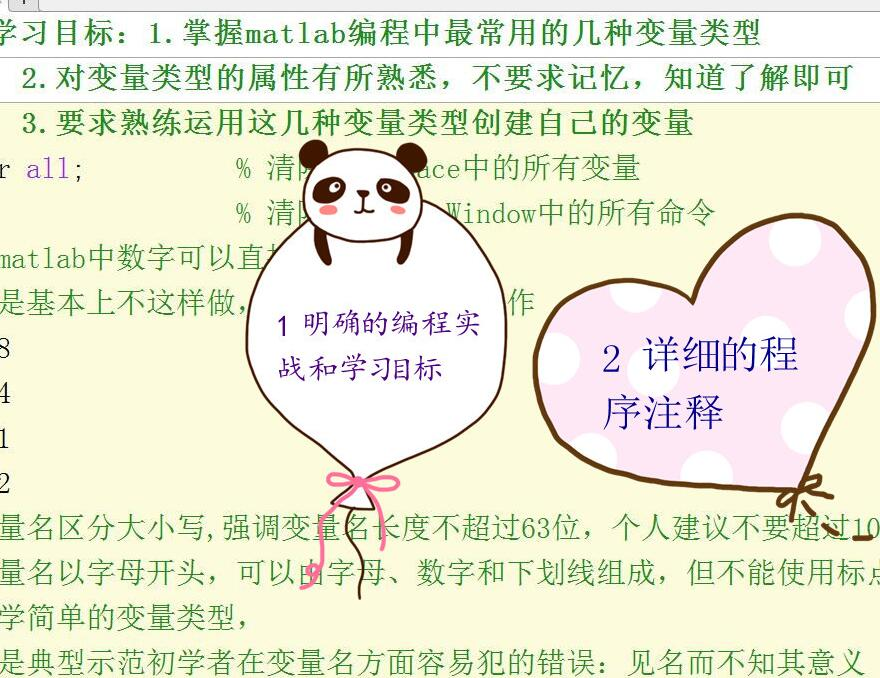「课程学习目录」

1.精通MATLAB图像处理常用技巧  2.Sobel等算子图像边缘检测matlab案例  3.matlab直线识别拟合角平分线和图像直线边缘提取案例  4.hough变换radon变换实现图像直线检测及傅里叶等多种变换技术案例  5.matlab边界跟踪算法和图像边界提取和图像减法除法去除图像背景  6.matlab直方图阈值法、区域、otsu图像分割案例和滤波、通过拉伸实现对比度等增强案例  7.matlab小波技术图像处理专题案例集

「7项超值权益，保障学习质量」
大咖讲解
技术专家系统讲解传授编程思路与实战。
答疑服务
专属社群随时沟通与讲师答疑，扫清学习障碍，自学编程不再难。
课程资料+课件
超实用资料，覆盖核心知识，关键编程技能，方便练习巩固。（部分讲师考虑到版权问题，暂未上传附件，敬请谅解）
常用开发实战
企业常见开发实战案例，带你掌握Python在工作中的不同运用场景。
大牛技术大会视频
2019Python开发者大会视频免费观看，送你一个近距离感受互联网大佬的机会。
APP+PC随时随地学习
满足不同场景，开发编程语言系统学习需求，不受空间、地域限制。

「什么样的技术人适合学习？」
想进入互联网技术行业，但是面对多门编程语言不知如何选择，0基础的你掌握开发、编程技术单一、冷门，迫切希望能够转型的你想进入大厂，但是编程经验不够丰富，没有竞争力，程序员找工作难。

「悉心打造精品好课，2天学到大牛3年项目经验」
【完善的技术体系】
技术成长循序渐进，帮助用户轻松掌握
掌握Matlab知识，扎实编码能力
【清晰的课程脉络】
浓缩大牛多年经验，全方位构建出系统化的技术知识脉络，同时注重实战操作。
【仿佛在大厂实习般的课程设计】
课程内容全面提升技术能力，系统学习大厂技术方法论，可复用在日后工作中。

「你可以收获什么？」
matlab边缘检测
matlab图像分割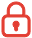订阅课程 开始学习


展开全文• 问题1：编写程序实现一阶Sobel算子，进行图像边缘提取； 问题2：编写程序实现一阶Prewitt算子，进行图像边缘提取； 问题3：编写程序实现一阶Roberts算子，进行图像边缘提取； 问题4：编写程序实现二阶...
• 多种边缘检测算子（Prewitt算子，LoG算子，Canny算子等）实现图像边缘检测分割
• 1、 编程实现图像阈值分割（阈值可以指定3个，分析分割结果，要理解阈值确定的基本方法）； 2、 Matlab边缘提取函数的应用。
•编程语言
• 基于Matlab图像分割和边缘检测教学的研究.pdf
• 更多MATLAB图像处理视频请点击  http://study.163.com/course/courseMain.htm?courseId=1003594013      所谓边缘是指其周围像素灰度有变化的那些像素的集合。边缘广泛存在于物体与...


更多MATLAB图像处理视频请点击  http://study.163.com/course/courseMain.htm?courseId=1003594013
所谓边缘是指其周围像素灰度有变化的那些像素的集合。边缘广泛存在于物体与背景之间、物体与物体之间、基元与基元之间。物体的边缘是由灰度不连续所反映的。基于边缘的分割代表了一大类基于图像边缘信息的方法，常用于图像分割。
更多MATLAB图像处理视频请点击  http://study.163.com/course/courseMain.htm?courseId=1003594013

1.边缘检测的基本原理及常用边缘检测算子
边缘检测的实质是采用某种算法来提取出图像中对象与背景间的交界线。我们将边缘定义为图像中灰度发生急剧变化的区域边界。图像灰度的变化情况可以用图像灰度分布的梯度来反映，因此我们可以用局部图像微分技术来获得边缘检测算子。经典的边缘检测方法是对原始图像中像素的某小邻域来构造边缘检测算子。以下是对几种经典的边缘检测算子进行理论分析，并对各自的性能特点做出了比较和评价。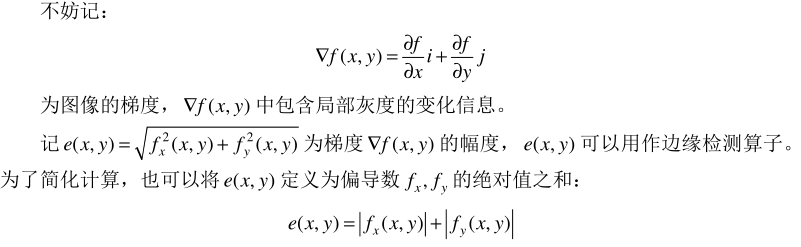人们以这些理论为依据，提出了许多算法，其中比较常用的边缘检测方法有差分边缘检测、Roberts 边缘检测算子、Sobel 边缘检测算子、Prewitt 边缘检测算子、Robinson 边缘检测算子、Laplace 边缘检测算子、Canny 算子和 LOG 算子等等。
（1）差分边缘检测方法
利用像素灰度的一阶导数算子在灰度迅速变化处得到高值来进行奇异点的检测。它在某一点的值就代表该点的“边缘强度”，可以通过对这些值设置阈值来进一步得到边缘图像。然而，用差分检测边缘必须使差分的方向与边缘方向垂直，这就需要对图像的不同方向都进行差分运算，增加了实际运算的繁琐性。
一般为垂直边缘、水平边缘、对角线边缘检测，如下所示：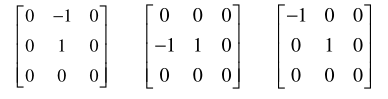差分边缘检测方法是最原始、最基本的方法。根据灰度迅速变化处一阶导数达到最大（阶跃边缘情况）原理，要求差分方向与边缘方向垂直，利用导数算子检测边缘。这种算子具有方向性，运算繁琐，目前很少采用。

（2）Roberts 边缘检测算子
Roberts 边缘检测算子根据任意一对互相垂直方向上的差分可用来计算梯度的原理，采用对角线方向相邻两像素之差，即：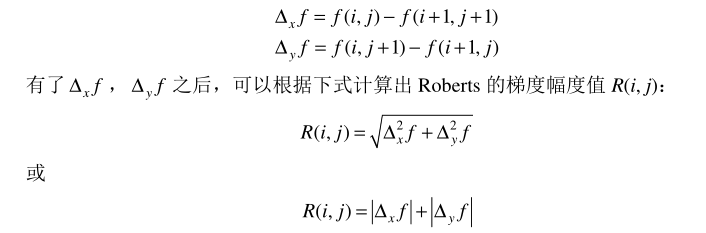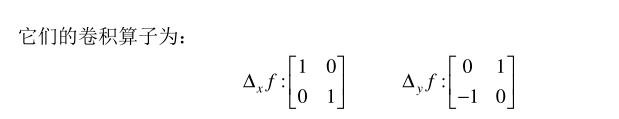适当选取门限 TH，并作如下判断：如果 R(i, j)＞TH，则(i, j)为阶跃状边缘点，{R(i, j)}为边缘图像。        Roberts 算子采用对角线方向相邻两像素之差近似梯度幅值检测边缘。检测水平和垂直边缘的效果好于斜向边缘，定位精度高，但对噪声敏感。
（3）Sobel 边缘检测算子
数字图像{f (i, j)}的每个像素，考察它上下左右邻点灰度的加权差，与之接近的邻点的权大。据此，定义 Sobel 算子如下：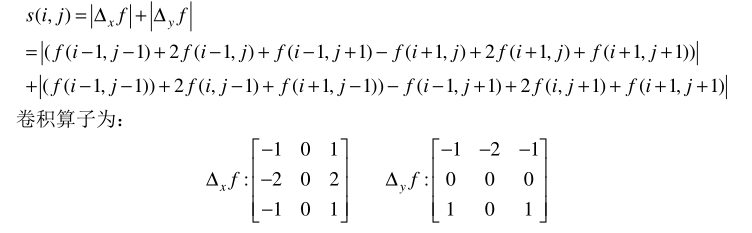适当选取门限 TH，并作如下判断：如果 R(i, j)＞TH，则(i, j)为阶跃状边缘点，{R(i, j)}为边缘图像。           Sobel 算子很容易在空间上实现，Sobel 边缘检测器不但产生较好的边缘检测效果，而且受噪声的影响也比较小。当使用大的邻域时，抗噪性能会更好，但这样会增加计算量，并且得出的边缘也会相应变粗。            Sobel 算子利用像素点上下、左右邻点的灰度加权算法，根据在边缘点处达到极值这一现象进行边缘的检测。Sobel 算子对噪声具有平滑作用，提供较为精确的边缘方向信息，但它同时也会检测出许多伪边缘，边缘定位精度不够高。当对精度要求不是很高时，它是一种较为常用的边缘检测方法。

（4）Prewitt 边缘检测算子
Prewitt 边缘检测算子是一种边缘样板算子。这些算子样板由理想的边缘子图像构成。依次用边缘样板去检测图像，与被检测区域最为相似的样板给出最大值。用这个最大值作为算子的输出值 P(i, j)，这样可将边缘像素检测出来。
定义 Prewitt 边缘检测算子模板如下：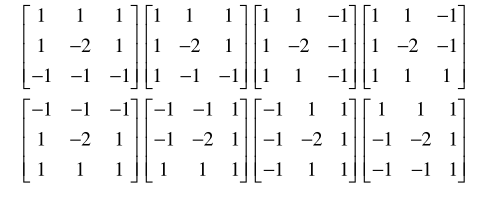８个算子样板所对应的边缘方向如图 所示。适当选取门限 TH，并作如下判断：如果 P(i,j)＞TH，则(i,j)为阶跃状边缘点，{P(i,j)}为边缘图像。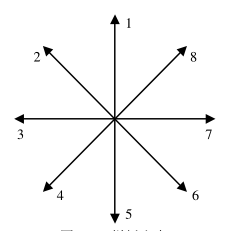（5）Robinson 边缘检测算子
Robinson 边缘检测算子也是一种边缘样板算子，其算法和 Prewitt 边缘检测算子相似，只是 8 个样板不同，如图所示。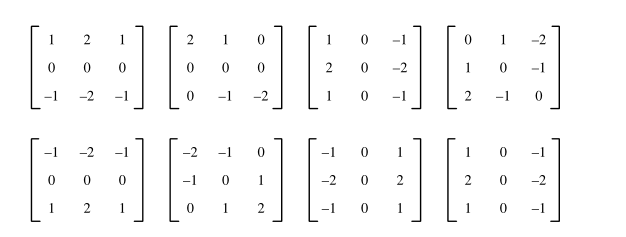（6）Laplace 边缘检测算子
Laplace 边缘检测算子是一种二阶微分算子，对于数字图像{ f (i,j)},它在图像中的位置(i,j)的 Laplace 定义如下：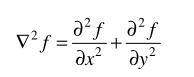Laplace 边缘检测算子是无方向性的算子，它比前面所述的多个方向导数算子的计算量要小，因为只用一个模板，且不必综合各模板的值。计算数字图像的 Laplace 值也是借助各种模板卷积实现的。实现 Laplace 运算的几种模板可见下图。在数字图像中，可用差分来近似微分运算，若选用图 7-4 中的第一个检测模板，则 f (i,j)的 Laplace 算子为：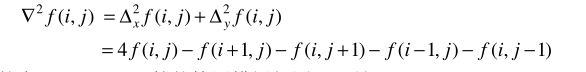几种常用的实现 Laplace 运算的检测模板如图所示。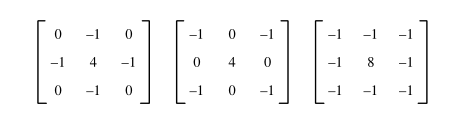由于 Laplace 算子是一种二阶导数算子，对图像中的噪声相当敏感。另外它常产生双像素宽的边缘，且也不能提供边缘方向的信息。由于以上原因，Laplace 算子很少直接用于检测边缘，而主要用于已知边缘像素后确定该像素是在图像的暗区或明区一边。

2.各种边缘检测算子的 MATLAB 实现及效果比较
在 MATLAB 中可以由 edge 函数实现各算子对边缘的检测，以 Roberts 算子为例，其语法格式如下：
BW=edge（I，’roberts’）      BW=edge（I，‘roberts’，thresh）      [BW，thresh]=edge（I，‘roberts’，⋯）      BW=edge（I，‘roberts’）自动选择阈值用 Robert 算子进行边缘检测。      BW=edge（I，‘roberts’，thresh）根据所指定的敏感阈值 thresh 用 Robert 算子进行边缘检测，它忽略了所有小于阈值的边缘。当 thresh 为空时，自动选择阈值
[BW，thresh]=edge（I，‘roberts’，⋯）返回阈值。
edge 函数对灰度图像 I 进行边缘检测，返回与 I 同样大的二值图像 BW，其中 1 表示边缘，0 表示非边缘。I 是 unit8 型、unit16 型，或者是 double 型，BW 是 unit8 型。
其余 Sobel 算子、Prewitt 算子、LOG 算子、Canny 算子的实现仅需将‘roberts’用‘sobel’、‘prewitt’、‘log’、‘canny’代替即可。
例：具体实现这几个算子的一个例程
I=imread('cameraman.tif'); BW1 = edge(I,'sobel'); %应用 Sobel 算子进行滤波 BW2 = edge(I,'roberts'); %应用 Roberts 算子进行滤波 BW3 = edge(I,'prewitt'); %应用 Prewitt 算子进行滤波 BW4 = edge(I,'log'); %应用 LOG 算子进行滤波 BW5 = edge(I,'canny'); %应用 Canny 算子进行滤波 subplot(2,3,1),imshow(I),title('原图'); >> subplot(2,3,2),imshow(BW1),title('Sobel算子边缘检测'); >> subplot(2,3,3),imshow(BW2),title('Roberts算子'); >> subplot(2,3,4),imshow(BW3),title('Priwitt算子'); >> subplot(2,3,5),imshow(BW4),title('LOG算子'); >> subplot(2,3,6),imshow(BW5),title('Canny算子');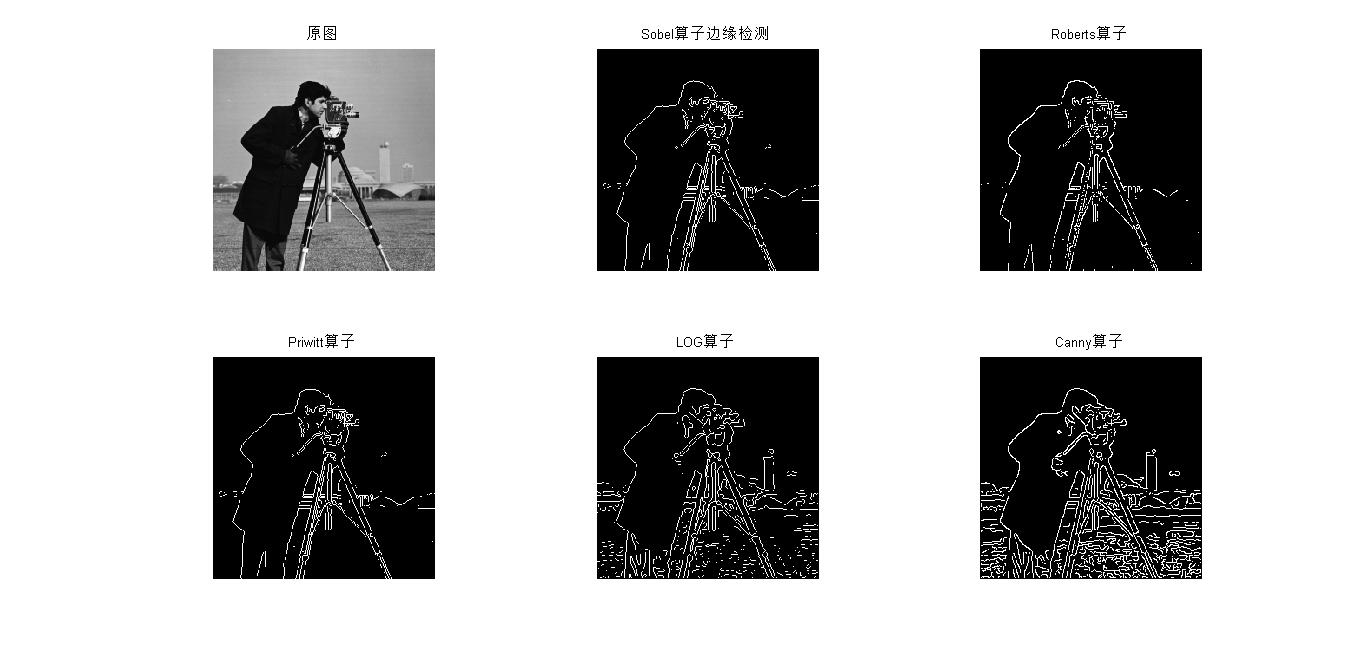更多MATLAB图像处理视频请点击  http://study.163.com/course/courseMain.htm?courseId=1003594013

展开全文• 数字图像处理中的拉普拉斯边缘检测算法，先计算图像直方图，灰度值分割阈值，并计算各阈值下的前景背景概率密度函数，再进行像素的累计熵
• ## 基于Matlab的图像分割----边缘检测

千次阅读 多人点赞 2020-12-15 20:34:35
在一幅图像中，人们往往只对其中的某些目标感兴趣，而这些目标通常占据一定的区域，并且在某些特性上（如灰度，轮廓，颜色及纹理等）上周围的图像有差别。这些差别可能很明显也可能很微小，随着计算机图像处理技术...
在一幅图像中，人们往往只对其中的某些目标感兴趣，而这些目标通常占据一定的区域，并且在某些特性上（如灰度，轮廓，颜色及纹理等）上和周围的图像有差别。这些差别可能很明显也可能很微小，随着计算机图像处理技术的发展，使得人们可以通过计算机来对图像进行某些方面的处理。其中，生活中最常见的就是图像识别技术（面部识别，指纹识别等），而图像识别的基础就是图像分割。所以讲，图像分割是后续高级图像处理学习的基础。
1.图像分割的基本知识
图像分割，就是图像中反应物体真实情况的，占据不同区域的，具有不同特性的目标区分开来，并形成数字特征。在现实生活的不同领域内，图像分割有着不同的叫法，但其核心是相同的。
1.1图像分割简单定义
(1)定义
图像分割是指将一幅图像分解为若干互不交叠的，有意义的，具有相同性质的区域。 (2)优秀的图像分割应具有的特点
a.分割出来的各区域对某种特性（比如灰度）而言具有相似性，区域的内部是连通的，且没有过多小孔； b.相邻区域对分割所依据的性质有明显的差异； c.区域的边界是明显的。
在实际的区域分割算法中，只能寻求较为满足上述特点的方法。因为，若是过于强调同一区域内性质一致性，则分割区域会产生很多小孔和一些不规整的边界；若是过于强调相邻区域显著的差异，则有很多分割区域就会合并到一起。所以，不同的分割技术总是在各种特点中寻求一种平衡。
(3)图像分割算法的共性
图像分割是图像处理和计算机视觉方便的经典难题，至今没有统一的标准进行评判，因此每年都会涌现出很多分割技术。然而，它们大多数遵循图像在像素级的两个性质：相似性和不连续性。属于同一目标的区域具有相似性，属于不同目标的区域在边界出现不连续性。
2.边缘检测
人们可能最先研究的就是基于边缘的图像分割方法，基于在图像的边缘灰度变化的往往比较剧烈，它试图通过检测图像中不同区域的边缘来实现对图像的分割。
2.1边缘检测概述
(1)概述
边缘检测技术非常重要，因为边缘时所要提取目标与背景的分界线，提取出边缘才能将目标与背景分隔开。 在图像中，边缘往往表征一个特征区域的终结和另一个特征区域的开始，边界所分开区域的内部特征或属性是一致的，而不同区域内部的特征或属性是不同的，边缘检测正是利用物体和背景在某种图像特征上的差异来实现的，这些差异包括灰度，颜色，纹理等。 边缘检测的实质就是检测图像特性发生变化的位置。 (2)基本内容
由于图像中噪音和模糊的存在，检测的边界很可能变宽或在某些点发生间断。因此，边缘检测包括两大基本内容： **a.**抽取出反映灰度变化的边缘点； **b.**剔除某些边界点或填补边缘间断点，并将这些边缘连接成线。 (3)边缘的基本知识
a.边缘
是指图像中像素灰度有阶跃变化或屋顶状变化的那些像素的集合。 b.边缘分类
1.阶跃状边缘：阶跃状边缘位于两边的像素灰度值有明显不同的地方（跳变后保持不变）； 2. 屋顶状边缘：屋顶状边缘位于灰度值从增加到减少的转折处（跳变后又恢复原状）。 阶跃状和屋顶状边缘其邻近灰度方向导数变化情况，见下图：2.2常见边缘检测算子
边缘检测算法中，通过边缘检测算子来检测图像的边缘是最为简单，也是最为经典的边缘检测方法。其基本思想是通过考察图像的每个像素点在某个邻域内灰度的变化，利用边缘邻近的一阶或二阶导数变化规律来判断该像素是否在图像区域的边界上。
2.2.1梯度算子检测边缘
最为简单的边缘检测算子就是梯度边缘算子，掌握了其边缘检测的方法，余下较复杂的边缘检测方法便能迎刃而解了。下面着重介绍利用梯度边缘算子检测边缘的算法。
(1)梯度定义式及简化计算 函数f(x,y)在(x,y)处的梯度为一个向量：计算这一向量的大小：模板表示：(2)检测边缘点步骤
计算梯度图像；
2）选取适当的阈值T，对梯度图像进行二值化； 当Grad[f (x，y)]≥T时，令f (x，y)＝l，则为阶跃状边缘点。否则f (x，y)＝0，形成一幅边缘二值图像。 (3)举例
源代码：
%1.边缘检测
%（1）梯度算子法
if size(mImg,3)>1%判读入图像是否为灰度图，不是，则转化成灰度图
mImg = rgb2gray(mImg);
end
mImg = im2double(mImg);

mBlock = [-1 1];%梯度算子

mImgDy = imfilter(mImg, mBlock, 'replicate');%滤波函数imfilter
mImgDx = imfilter(mImg, mBlock','replicate');
mImgDxy = sqrt(mImgDy.*mImgDy+mImgDx.*mImgDx);

figure;
subplot(221), imshow(mImg),title('灰度图');
subplot(222), imshow(mat2gray(abs(mImgDy))),title('横向偏导后图像');
subplot(223), imshow(mat2gray(abs(mImgDx))),title('纵向偏导后图像');
subplot(224), imshow(mat2gray(mImgDxy)),title('梯度图');

vThred = 0.3;%设定阈值
mImgDxy = mat2gray(mImgDxy);%根据阈值将灰度图转化成二值图像，以便更好地体现出边缘
mImgDxy(mImgDxy<vThred) = 0;
mImgDxy(mImgDxy>=vThred) = 1;
mImgDxy = bwmorph(mImgDxy, 'thin');
figure,imshow(mImgDxy),title('二值图像');

其中，若对函数imfilter有兴趣，请参见imfilter函数详解。
运行结果：(3)效果分析
梯度算子仅用最近邻像素的灰度计算，对噪声敏感，无法抑制噪声的影响。
2.2.2其他边缘检测算子
其他常见的边缘检测算子还有：罗伯特（Roberts）边缘算子，索贝尔（Sobel）边缘算子，Prewitt边缘算子，拉普拉斯（Laplacian）边缘算子，方向（Kirsch）算子，坎尼（Canny）边缘算子。
(1)Roberts边缘算子
Roberts边缘算子是一种斜向偏差分的梯度计算方法，梯度的大小代表边缘的强度，梯度的方向和边缘走向垂直。 a.差分表达式b.模板表示c.Roberts近似计算d.处理效果
Roberts边缘算子也不能很好的消除噪声的影响，但效果还是较梯度好。
(2)Prewitt边缘算子
a.算子思想
在检测边缘的同时减少噪声的影响，Prewitt从加大边缘检测算子的模板大小出发，由2×2扩大到3×3来计算差分算子。
b.模板表示c.处理效果
Prewitt算子检测边缘点的同时能抑制噪声的影响。
(3)Sobel边缘算子
a.算子思想
Sobel在Prewitt算子的基础上，对4—邻域采用带权的方法计算差分。 b.模板表示c.处理效果
Sobel算子检测边缘点的同时能进一步抑制噪声的影响，但检测的边缘较宽。
d.举例
源代码：
%(2)sobel边缘算子
if size(mImg,3)>1
mImg = rgb2gray(mImg);
end
mImg = im2double(mImg);

mBlock = fspecial('sobel');

mImgDy = imfilter(mImg, mBlock, 'replicate');
mImgDx = imfilter(mImg, mBlock','replicate');
mImgDxy = sqrt(mImgDy.*mImgDy+mImgDx.*mImgDx);

figure;
subplot(121), imshow(mImg),title('灰度图');
subplot(122), imshow(mat2gray(mImgDxy)),title('sobel梯度图');

运行结果：(4)Kirsch边缘算子
a.算子思想
利用一组模板分贝计算不同方向的差分值，取其中的最大值作为边缘强度，而将与之对应的方向作为边缘方向。
b.模板表示
Kirsch（3×3）八方向模板:各方向间的夹角为45°，见下图。(5)Laplacian边缘算子
a.算子定义
二维函数的拉普拉斯变换是一个二阶的微分定义：其差分形式有很多种表示形式，最常见的是：b.模板表示
拉普拉斯算子对应的模板形式要求，作用于中心像素的系数是一个正数，而且其周围像素的系数为负数，系数之和必为0。举例如下：c.处理效果
优点 ：各向同性，对细线和孤立点检测效果好（可以理解一下）。
缺点：对噪音敏感，会产生双像素的边缘，不能检测出边的方向。
适用场合：拉普拉斯算子不直接用于边的检测，通常只起第二位的角色。即检测一个像素是在边的亮的一边还是暗的一边；利用零跨越，确定边的位置。
注意事项：由于Laplacian算子和梯度算子一样都对噪声比较敏感，且Laplacian算子对噪声还有加强的作用，因此在实际的图像边缘检测中常常需要将待检测的图像先进行平滑除噪。
d.实例
源代码：
%(4)Laplacian算子边缘检测
if size(mImg,3)>1
mImg = rgb2gray(mImg);
end
mImg = im2double(mImg);
mImg = medfilt2(mImg,[5 5]);%中值滤波

mBlock = fspecial('laplacian')/8;

mImgDxy = imfilter(mImg, mBlock,'replicate');
mImgDxy = abs(mImgDxy);

subplot(121),imshow(mImg),title('灰度图');
subplot(122),imshow(mat2gray(mImgDxy)),title('Laplacian算子检测后的图像');

运行结果：(6)Canny边缘算子
坎尼(Canny)算子检测法是一类比较成熟的边缘检测算子法中完善的边缘检测方法，因此，在实际的边缘检测实例中，坎尼算子检测方法是很常见的。
a.基本思想
先对图像选择一定的高斯滤波器进行平滑滤波，抑制图像中的噪声；然后求取梯度图像；接着采用一种“非极大值抑制”的技术，细化边缘；最后利用双门限制用两个阈值递归寻找边缘点，以实现边缘检测。
b.一般步骤
对图像进行高斯滤波平滑；求取梯度图像；对梯度幅值应用非极大值抑制，其过程为找出图像梯度中的局部极大值点，把其他非局部极大值点置零以得到细化的边缘；滞后阈值化：使用两个阈值T1和T2（T1＞T2），使得噪声造成的中断可以被连接。
c.独有特点
Canny算子与其他算子最大的不同体现在，其分别采用了两个不同的阈值对图像中的强边缘和弱边缘进行检测，并且当且仅当弱边缘和强边缘有连接时，才在最后的检测结果中将弱边缘显示出来。
d.Canny检测实例图片2.3边缘检测函数介绍
在Matlab图像处理的工具箱中，edge函数提供了部分检测边缘的算法，详细参见Matlab中edge函数使用方法或者在matlab中查看帮助文档。
3.区域分割
一幅图像中属于同一区域的像素应具有相同的或相似的属性，不同区域的像素属性不同。因此，区域分割就是把相同属性归属同一区域的过程。
3.1阈值分割
当只利用一个属性进行分割时，区域分割就变成确定属性的阈值的问题。在数字图像处理中， 我们把只具有两类区域的图像称为最简单图像。
3.1.1阈值分割的定义
1.数学定义
设原始图像f(x,y),按照一定规则确定了一组阈值T1,T2,T3…Tn,其中n >= 1,将原始图像分割成几部分，分割过后的图像为：2.阈值的类型
一般情况下，阈值运算可以看成针对图像中某一像素点的灰度，该点的某种局部特征以及该点的位置的一种函数，这种阈值函数可以表示为：
T(x,y,N(x,y),f(x,y)) 其中，f(x,y)是该点的灰度值，N(x,y)是该点的局部邻域特征。根据对T的不同约束程度，可得到三种阈值：
（1）全局阈值：T = T(f(x,y)),即阈值只与点的灰度有关； （2）局部阈值：T = T(N(x,y),f(x,y)),即阈值和点的灰度和局部邻域特征有关； （3）动态阈值：T = T(x,y,N(x,y),f(x,y)),即阈值和点的位置，灰度值以及局部邻域特征都有关系。
3.阈值分割类型和假设
a.类型
根据选择阈值个数进行划分，阈值分割可分为单阈值分割与多阈值分割。
b.阈值分割的基本假设
全局阈值分割后的同一区域内相邻像素间灰度值应具有相似性，不同目标区域的像素间的灰度应有明显的差异，反映在直方图上就是不同的区域对应着不同的峰。
3.1.2人工阈值选择方法
阈值分割实现简单，但如何去选择合适的阈值这对最终的处理效果影响很大。其中最简单的就是人工选择法，即人通过自己的观察，在分析直方图的基础上，去选择较为合适的阈值。
下面有两种基于对最简单图像阈值分割的人工判断策略：
（1）状态法（峰谷法）（2）判断分析法3.1.3自动阈值选择方法
虽然人工法可以选择出令人满意的阈值，但是在无人为介入如选择合适的阈值是在很多应用中所需要的，为此这里简单介绍几种自动选择阈值的方法，迭代式阈值选择，Otsu法阈值选择法，最小误差阈值选择法。
3.1.4综合举例
源代码：
%2.区域分割---阈值分割
%（1）综合举例
image = rgb2gray(image);
image = im2double(image);   %类型转换
%全局阈值
Th = 0.5 * (min(image(:)) + max(image(:)));
done = false;
while ~done      %迭代处理
indc = image >= Th;     %indc为索引值
Tn = 0.5 * (mean(image(indc)) + mean(image(~indc)));%Tn为迭代阈值
done = abs(Th - Tn) < 0.1;
Th = Tn;
end
display('Threshold(Th)---Iterative');%在命令窗口显示文字  iterative(迭代)
Th
image1 = im2bw(image,Th);%根据阈值Th将图像转化为二值图像
subplot(221),imshow(image),title('Original image');
subplot(222),imshow(image1),title('Global interative processing image');
Ts = graythresh(image);%Global image threshold using Otsu's method
display('Threshold(Ts)---Otsu method ');
Ts
image2 = im2bw(image,Ts);
subplot(223),imshow(image2),title('Global Otsu method processing image');
se = strel('disk',10);%生成形态结构元素
image3 = imtophat(image,se);%顶帽过滤
Tl = graythresh(image3);%生成局部阈值Tl
display('Threshold(Tl)---Local thresholding');
Tl
image4 = im2bw(image,Tl);
subplot(224),imshow(image4),title('Global local threshold processing image');

运行结果：
figure窗口：命令行窗口：3.2分水岭算法
3.2.1基本思想
分水岭算法是一种借鉴了形态学图像处理的算法，在该方法中，将图像看成一个拓扑地形图，其中灰度值f(x,y)对应地形高度图。高灰度值对应着山峰，地灰度值对应着山谷。水总是朝地势低的地方流动，直到在某一局部低洼处才会停下来，此低洼处称作吸水盆地，最终水会分聚在不同的吸水盆地中，吸水盆地之间的山脊就叫做分水岭。水从分水岭流过时，它朝不同的吸水盆地流向的可能性是相同的。于是，将这种思想应用到图像分割中，就是要在灰度图像中找到不同的“吸水盆地”和“分水岭”，而这些分水岭和吸水盆地就组成了我们要分割得到的区域。
在Matlab中，提供了函数**watershed**可用于显示分水岭算法。
3.2.2应用举例
下面通过一个简单的例子直观感受一下，分水岭算法的处理效果。
例子：使用距离变换和分水岭算法实现对图像分割
源代码：
%3.分水岭算法实现图像分割
%(1)分水岭算法
bw = im2bw(f,graythresh(f));%转化成二值图像
bwc = ~bw;%图像反色
dst = bwdist(bwc);%二值图像距离变换
% 计算二值图像BW的欧氏距离变换。对于BW中的每个像素，距离变换指定一个数字
% 即该像素与BW中最近的非零像素之间的距离。
ws = watershed(-dst);
w = ws==0;
rf = bw&~w;
figure;
subplot(221),imshow(f),title('原图像');
subplot(222),imshow(bw),title('二值图像');
subplot(224),imshow(rf),title('距离变换与二值图像结合图');
subplot(223),imshow(ws),title('分水岭距离变换的图像');
subplot(224),imshow(rf),title('距离变换与二值图像结合图');

h = fspecial('sobel');%生成sobel滤波模板
fd = im2double(f);%类型转换
sq = sqrt(imfilter(fd,h,'replicate').^2 + imfilter(fd,h','replicate').^2);%求取sobel梯度模板处理后的梯度幅值
sqoc = imclose(imopen(sq,ones(3,3)),ones(3,3));%图像闭合运算
wsd = watershed(sqoc);
wg = wsd == 0;
rfg = f;
rfg(wg) = 255;
figure;
subplot(221),imshow(wsd),title('分水岭梯度图');
subplot(222),imshow(rf),title('距离变换与二值图像结合图');
im = imextendedmin(f,20);%扩展极小值变换,输出是二值图像
Lim = watershed(bwdist(im));
subplot(223),imshow(Lim),title('分水岭标记控制');
em = Lim == 0;
rfmin = imimposemin(sq,im|em);
% I2=imimposemin（I，BW）使用形态学重建来修改强度图像I，因此它只有当BW
% 不为零时的区域最小值。BW是一个与I大小相同的二进制图像。
wsdmin = watershed(rfmin);
subplot(224),imshow(rfmin),title('分水岭梯度与标记控制结合图');
rfgm = f;
rfgm(wsdmin == 0) = 255;
figure;
imshow(rfgm),title('分水岭算法处理过后分割的图像');

运行结果：相关函数补充：
1.分水岭算法函数：watershed； 2.二值图像距离变换函数：bwdist; 3.形态学处理函数：imclose与imopen; 4.扩展极小值变换函数：imextendedmin; 5.形态重构修改强度图像函数：imimposemin.
4.区域增长
4.1基本思想
区域增长是从图像的一个像元或一个邻域出发，比较相邻部分的特征，按照事先定义好了的相似原则不断合并直到不能合并为止的分割方法。也称作区域扩张。 区域增长处理的基本任务： 1）确定区域的数目； 2）选择有判断意义的特征； 3）确定相似性准则。
其中，确定相似性准则最为重要。
4.2常见增长算法
根据邻域性质和相似性准则的不同，常见的区域增长算法可分为：
1）单一型区域增长-------------像素与像素；
2）质心型区域增长-------------像素与区域；
3）混合型区域增长-------------区域与区域。
下面详细介绍这三种策略：
1.单一型区域增长
单一型区域增长是像素级的区域增长，又称为简单图像扩张法，详细参见如下：上图的例子中，选择起始灰度值为‘9’的像素点，与它周围的像素点进行比较，若灰度差值小于设定的阈值T = 1.5，这将该相邻像素点的灰度值设为‘9’，反之，不变，以此类推，扫描整个图像区域。
处理特点：方法简单，但如果区域之间的边缘灰度变化很平缓时，两个区域会合并起来（致命缺点）。2.质心型区域增长
质心型增长则略微改善了简单区域扩张法，它是使用已存在区域的像素灰度平均值与邻像素灰度值进行比较。其它步骤与上述相同。
缺点：区域增长的结果非常依赖起始像素点，当起始像素点不同时，区域增长的结果会有很大差异。3.混合型区域增长
a.基本思想
将图像分成若干小块，根据相似准则比较每一小块，若它们之间满足相似性条件，则合并到一块。
b.处理步骤
1）设灰度差的阈值为0，用单一型增长合并像素，得到初始分割图像（简单分块处理）；
2）从一个小区域开始，比较相邻区域的灰度差，将最小的相邻区域合并（比较合并）；
3）重复步骤2。
c.特点
优点：不依赖于起点；缺点：必须指定合并次数，否则整幅图像将被合并。
d.常用的相似性判断准则
根据区域内的灰度分布相似性进行合并。
步骤：注：上图直方图为累积直方图。
展开全文计算机视觉 算法
• Matlab边缘检测和区域生长图像分割算法代码-deer.m 我的毕业设计，顺利通过。希望对朋友们有帮助。
• clc; clear all; close all; I=im2double(imread('D:\Gray Files\10-26.tif'));...%=============================边缘检测(五)================================= % Canny Edge Detector %------------...
clc;
clear all;
close all;
[M,N]=size(I);
%%
%=============================边缘检测(五)=================================
% Canny Edge Detector
%-------------------------用高斯低通滤波器平滑图像-------------------------
%建筑图像所设参数
% n=25;
% sigma=4;
%头颅扫描图像所设参数
n=13;
sigma=2;
%lena测试图像所设参数
% n=5;
% sigma=1;
type='symmetric';
f_s=GaussianBlur(n,I,sigma,type);
% imshow(f_s)
% imwrite(f_s,'D:\Gray Files\lena-test.jpg','jpg');
%-----------------------计算平滑后的图像梯度和角度-------------------------
n_l=1;
%Sobel算子
s_y=[-1 -2 -1;
0 0 0;
1 2 1];
s_x=[-1 0 1;
-2 0 2;
-1 0 1];
%定义梯度和角度
gx=zeros(M,N);
gy=zeros(M,N);
for i=1:M
for j=1:N
gx(i,j)=sum(sum(Block.*s_x));
gy(i,j)=sum(sum(Block.*s_y));
end
end
type='replicate';
gx=GaussianBlur(n,gx,sigma,type);
gy=GaussianBlur(n,gy,sigma,type);
M_s=sqrt(gx.^2+gy.^2);
M_s=M_s/max(M_s(:));
a_s=atan2(gy,gx)*180/pi;
% imshow(M_s)
%-----------------------对梯度图像进行非极大值抑制-------------------------
n_l=1;
%定义非极大值抑制图像
g_N=M_s;
for i=1:M
for j=1:N
%取出中心点的梯度值

theta=a_s(i,j);
if (theta>=0 && theta<=45) ||...
(theta<-135 && theta>=-180)
K1=(yBot(2)-yBot(1))*k+yBot(1);
K2=(yTop(2)-yTop(1))*k+yTop(1);
end
if (theta>45 && theta<=90) ||...
(theta<-90 && theta>=-135)
K1=(yBot(2)-yBot(1))*k+yBot(1);
K2=(yTop(2)-yTop(1))*k+yTop(1);
end
if (theta>90 && theta<=135) ||...
(theta<-45 && theta>=-90)
K1=(yBot(2)-yBot(1))*k+yBot(1);
K2=(yTop(2)-yTop(1))*k+yTop(1);
end
if (theta>135 && theta<=180) ||...
(theta<0&& theta>=-45)
K1=(yBot(2)-yBot(1))*k+yBot(1);
K2=(yTop(2)-yTop(1))*k+yTop(1);
end
if K<K1 || K<K2
g_N(i,j)=0;
end
end
end
g_N=g_N/max(g_N(:));
imshow(g_N)

%-------------------------------设置双门限---------------------------------
%对非极大值抑制图像进行扩展(0)，方便后续的边界处理
n_l=1;
%lena图像所设参数
% H=0.275;
% L=0.25;
%高门限
% T_H=T_max*H;
%低门限
% T_L=T_H*L;
%-------头颅图像所设参数
T_H=0.15;
T_L=0.05;
%------建筑图像所设参数
% H=0.1;
% L=0.04;
% %高门限
% T_H=T_max*H;
% %低门限
% T_L=T_H*L;

%寻找大于高门限点

%--------------------------消除未连通的边界点------------------------------
M_temp=M+2;
g_N_copy(ind_H)=0;
ind_L=find(g_N_copy~=0);
while ~isempty(ind_H)
%取出第一个点
p=ind_H(1,1);
%初始化连通点集
Conn=[];
%查找p点是否有连通点，即在ind_L中是否有值
[ind_L,Conn]=FindConnected_8(p,ind_L,Conn,M_temp);
%向下查找所有与p点连通的点
while ~isempty(Conn)
%从连通集中取出第一个点，而后删除该点
p1=Conn(1,:);
Conn(1,:)=[];
[ind_L,Conn]=FindConnected_8(p1,ind_L,Conn,M_temp);
end
%标记p点已访问，即从ind_H中删除
ind_H(1,:)=[];
end
%将ind_L中未与ind_H连通的点，在图像中置零
% imshow(g_N)
%-----------------------------图像细化处理---------------------------------
[g]=ImageThinning(g_N);
imshow(g)
高斯模糊GaussianBlur函数：
function [g]=GaussianBlur(n,I,sigma,type)
[M,N]=size(I);
%生成高斯核函数
G=GaussianKernelG(n,sigma);
%平滑图像
n_l=n-1;
g=zeros(M,N);
%对原图进行扩展，方便处理边界
for i=1:M
for j=1:N
%获得图像子块区域
%用Kirsch内核对子区域卷积
g(i,j)=sum(sum(Block.*G));
end
end
%归一化
g=g/max(g(:));
end
%生成高斯核函数
% n 核函数的大小
function G=GaussianKernelG(n,sigma)
n_l=floor(n/2);
%初始化
G=zeros(n,n);
%产生高斯核矩阵
for i=-n_l:n_l
for j=-n_l:n_l
d=i^2+j^2;
G(i+n_l+1,j+n_l+1)=exp(-(d)/(2*sigma^2));
end
end
%寻找最小值
m=sum(G(:));
%取整
G=G/m;
%将大于3*delta的取值置零
for i=-n_l:n_l
for j=-n_l:n_l
d=sqrt(i^2+j^2);
if d>3*sigma
G(i+n_l+1,j+n_l+1)=0;
end
end
end
end
查找8连通函数，FindConnected_8：
%查找所有8连通的点
% p为中心点，ind_L为有效矩阵,Conn为连通矩阵，M为图像矩阵的行数
function [ind_L,Conn]=FindConnected_8(p,ind_L,Conn,M)
%查找p点是否有连通点，即在ind_L中是否有值
%上
c=find(ind_L==p-1);
if ~isempty(c)
Conn=cat(1,Conn,p-1);
ind_L(c)=[];
end
%下
c=find(ind_L==p+1);
if ~isempty(c)
Conn=cat(1,Conn,p+1);
ind_L(c)=[];
end
%左
c=find(ind_L==p-M);
if ~isempty(c)
Conn=cat(1,Conn,p-M);
ind_L(c)=[];
end
%左上
c=find(ind_L==p-M-1);
if ~isempty(c)
Conn=cat(1,Conn,p-M-1);
ind_L(c)=[];
end
%左下
c=find(ind_L==p-M+1);
if ~isempty(c)
Conn=cat(1,Conn,p-M+1);
ind_L(c)=[];
end
%右
c=find(ind_L==p+M);
if ~isempty(c)
Conn=cat(1,Conn,p+M);
ind_L(c)=[];
end
%右上
c=find(ind_L==p+M-1);
if ~isempty(c)
Conn=cat(1,Conn,p+M-1);
ind_L(c)=[];
end
%右下
c=find(ind_L==p+M+1);
if ~isempty(c)
Conn=cat(1,Conn,p+M+1);
ind_L(c)=[];
end

end
图像细化函数，ImageThinning如下：
%图像细化,目前只对二值图像进行处理
function [g]=ImageThinning(I)
n_l=1;
%对边界图进行扩充，四周各加1行、1列0（与结构元素的大小相对应），目的是为了处理边界点
%获得扩充图像大小
%寻找图像中的亮点，即值为1的点
ind_c=[];
while ~isequal(ind_c,ind)
%备份赋值，以便下一次循环开始进行比较
ind_c=ind;
%保存ind中符合条件的下标
ind_sub=[];
%按照B1结构元素搜索
for i=1:length(ind)
p=ind(i,1);
if ~isempty(find(ind==p+1)) && ~isempty(find(ind==p-M+1)) && ~isempty(find(ind==p+M+1)) &&...
isempty(find(ind==p-1)) && isempty(find(ind==p-M-1)) && isempty(find(ind==p+M-1))
ind_sub=cat(1,ind_sub,i);
end
end
%将下标符合条件的数值，从ind中清除，以下类似
if ~isempty(ind_sub)
ind(ind_sub)=[];
end
ind_sub=[];
%按照B2结构元素搜索
for i=1:length(ind)
p=ind(i,1);
if ~isempty(find(ind==p+1)) && ~isempty(find(ind==p-M)) && ~isempty(find(ind==p-M+1)) &&...
isempty(find(ind==p-1)) && isempty(find(ind==p+M)) && isempty(find(ind==p+M-1))
ind_sub=cat(1,ind_sub,i);
end
end
if ~isempty(ind_sub)
ind(ind_sub)=[];
end
ind_sub=[];
%按照B3结构元素搜索
for i=1:length(ind)
p=ind(i,1);
if ~isempty(find(ind==p-M-1)) && ~isempty(find(ind==p-M)) && ~isempty(find(ind==p-M+1)) &&...
isempty(find(ind==p+M-1)) && isempty(find(ind==p+M)) && isempty(find(ind==p+M+1))
ind_sub=cat(1,ind_sub,i);
end
end
if ~isempty(ind_sub)
ind(ind_sub)=[];
end
ind_sub=[];
%按照B4结构元素搜索
for i=1:length(ind)
p=ind(i,1);
if ~isempty(find(ind==p-1)) && ~isempty(find(ind==p-M)) && ~isempty(find(ind==p-M-1)) &&...
isempty(find(ind==p+1)) && isempty(find(ind==p+M)) && isempty(find(ind==p+M+1))
ind_sub=cat(1,ind_sub,i);
end
end
if ~isempty(ind_sub)
ind(ind_sub)=[];
end
ind_sub=[];
%按照B5结构元素搜索
for i=1:length(ind)
p=ind(i,1);
if ~isempty(find(ind==p-M-1)) && ~isempty(find(ind==p-1)) && ~isempty(find(ind==p+M-1)) &&...
isempty(find(ind==p-M+1)) && isempty(find(ind==p+1)) && isempty(find(ind==p+M+1))
ind_sub=cat(1,ind_sub,i);
end
end
if ~isempty(ind_sub)
ind(ind_sub)=[];
end
ind_sub=[];
%按照B6结构元素搜索
for i=1:length(ind)
p=ind(i,1);
if ~isempty(find(ind==p-1)) && ~isempty(find(ind==p+M-1)) && ~isempty(find(ind==p+M)) &&...
isempty(find(ind==p+1)) && isempty(find(ind==p-M+1)) && isempty(find(ind==p-M))
ind_sub=cat(1,ind_sub,i);
end
end
if ~isempty(ind_sub)
ind(ind_sub)=[];
end
ind_sub=[];
%按照B7结构元素搜索
for i=1:length(ind)
p=ind(i,1);
if ~isempty(find(ind==p+M-1)) && ~isempty(find(ind==p+M)) && ~isempty(find(ind==p+M+1)) &&...
isempty(find(ind==p-M-1)) && isempty(find(ind==p-M)) && isempty(find(ind==p-M+1))
ind_sub=cat(1,ind_sub,i);
end
end
if ~isempty(ind_sub)
ind(ind_sub)=[];
end
ind_sub=[];
%按照B8结构元素搜索
for i=1:length(ind)
p=ind(i,1);
if ~isempty(find(ind==p+1)) && ~isempty(find(ind==p+M)) && ~isempty(find(ind==p+M+1)) &&...
isempty(find(ind==p-1)) && isempty(find(ind==p-M)) && isempty(find(ind==p-M-1))
ind_sub=cat(1,ind_sub,i);
end
end
if ~isempty(ind_sub)
ind(ind_sub)=[];
end
end
%m连通检测
ind_c=[];
while ~isequal(ind_c,ind)
ind_c=ind;
ind_back=ind;
while ~isempty(ind_back)
p=ind_back(1,:);
%如果p点四联通中有三个值为1，则将该点置为零
if (~isempty(find(ind==p+1)) && ~isempty(find(ind==p+M)) && ~isempty(find(ind==p-M))) ||...
(~isempty(find(ind==p-1)) && ~isempty(find(ind==p+M)) && ~isempty(find(ind==p-M))) ||...
(~isempty(find(ind==p+1)) && ~isempty(find(ind==p-1)) && ~isempty(find(ind==p-M))) ||...
(~isempty(find(ind==p+1)) && ~isempty(find(ind==p-1)) && ~isempty(find(ind==p+M)))
c=find(ind==p);
ind(c)=[];

end
%如果p点四联通中有两个值为1，且其对角为0，则将该点置为零
if (~isempty(find(ind==p+1)) && ~isempty(find(ind==p+M)) && isempty(find(ind==p-M-1))) ||...
(~isempty(find(ind==p-1)) && ~isempty(find(ind==p+M)) && isempty(find(ind==p-M+1))) ||...
(~isempty(find(ind==p+1)) && ~isempty(find(ind==p-M)) && isempty(find(ind==p+M-1))) ||...
(~isempty(find(ind==p-1)) && ~isempty(find(ind==p-M)) && isempty(find(ind==p+M+1)))
c=find(ind==p);
ind(c)=[];
end
ind_back(1,:)=[];
end
end

%删除扩展的边缘
g(ind)=1;
g=g(2:M-1,2:N-1);
end

展开全文Canny算法
• 用于彩色图像边缘检测和分割的代码 ，matlab和c++混编的 刚从伯克利大学网站上下下来的 编译的时候遇到点问题 大家共同探讨
• MATLAB实现Canny图像边缘检测，此为本人研一时的图像处理与成像制导的作业，完全可以实现，并且附有详细的实验报告。
• 执行快速准确的亚像素边缘检测，基于改进的zernike矩方法，有需要的可以下载~~
• matlab实现灰度图像边缘检测，本代码采用roberts算子，其他算子代码方法类似！
• matlab 图像边缘检测 Marr算子 (源代码)
• %Edge linking using local processing clc; clear all; close all; %边缘连接测试图像 I=im2double(imread('D:\Gray Files\10-27.tif'));...%=============================边缘检测(六)=========================...
• 最近在学习MATLAB图像分割部分，现将几个边缘检测算法分享。 有sobel算法、prewitt算法、log算法 图1 图2 如图2所示，使用sobel算法处理rgb彩色图像 sobelBW1R为彩图R通道提取的边缘， sobelBW1G为G...
• 利用Matlab处理图像，使用几种方法进行边缘分割
• 为利用matlab进行图像处理实验的小部分代码，这部分代码非常简单，仅需直接调用内置函数即可完成，所以做成简单整理算是分享啦...

# matlab图像边缘检测和分割matlab 订阅